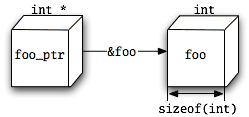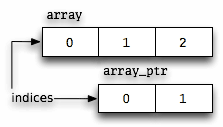# 你需要知道关于C语言指针的一切

Everything you need to know about pointers in C

(嗯，简短的段落。)

## 开始

int foo;

int *foo_ptr = &foo;

foo_ptr被声明为指向int的指针。我们已经初始化它指向foo。## 插曲：声明语法

int* ptr_a, ptr_b;

• 如果包含指向int的指针的变量的类型是int *，

* bzzt *错误！
ptr_b的类型为int。 它不是指针。
C的声明语法忽略指针星号时携带类型到多个声明。 如果你将ptr_a和ptr_b的声明分成多个声明，你会得到这样：

int *ptr_a;int ptr_b;

int *ptr_a, ptr_b;

int ptr_b, *ptr_a;

int *ptr_a, *ptr_b;

int ((not_a_pointer)), (*ptr_a), (((*ptr_b)));

## 赋值和指针

foo_ptr = 42;

（顺便说一下，编译器通常会在你试图为一个指针变量赋值时发出警告，gcc将会说“warning：initialization让指针从整型变为无转义”。）

## 解除引用

int bar = *foo_ptr;

*foo_ptr = 42; Sets foo to 42

（这被称为“存储”操作。）

## 插段：数组

int array[] = { 45, 67, 89 };

C的一个整洁的特性是，在大多数地方，当你再次使用名称数组，你实际上将使用指向它的第一个元素的指针（在C术语，＆array ）。这被称为“衰变”：数组“衰减”为指针。数组的大多数用法等于if数组已被声明为指针。

（如果“array”实际上是一个指针变量，那么它们并不意味着相同的事情，因为指针变量的地址不同于其中的地址 - 因此，中间表达式＆array不会等于另外两个表达式。只有当数组真的是一个数组时，三个表达式都是相等的。）

## 指针算术（或：为什么1 == 4）

int *array_ptr = array;printf(" first element: %i\n", *(array_ptr++));printf("second element: %i\n", *(array_ptr++));printf(" third element: %i\n", *array_ptr);

first element: 45second element: 67 third element: 89

## 索引

printf("%i\n", array);

45

int array[] = { 45, 67, 89 };int *array_ptr = &array;printf("%i\n", array_ptr);

89## 插曲：结构和联合

C中最有趣的两种类型是结构和联合。 您使用struct关键字创建一个结构类型，并使用union关键字创建联合类型。

struct foo {size_t size;char name;int answer_to_ultimate_question;unsigned shoe_size;};

struct foo my_foo;my_foo.size = sizeof(struct foo);

One way to do it(*foo_ptr).size = new_size;

Yummyfoo_ptr->size = new_size;

Icky(*foo_ptr_ptr)->size = new_size; One way(**foo_ptr_ptr).size = new_size; or another

Yummyfoo_ptr_ptr^^.size := new_size;

（但抛开这个抱怨，C是一个更好的语言。）

## 多级间接地址

int a = 3;int *b = &a;int **c = &b;int ***d = &c;

• *d == c; 解除引用an (int ***) once gets you an (int **) (3 - 1 = 2)
• **d == *c == b; 解除引用an (int ***) twice, or an (int **) once, gets you an (int *) (3 - 2 = 1; 2 - 1 = 1)
• ***d == **c == *b == a == 3; 解除引用 an (int ***) thrice, or an (int **) twice, or an (int *) once, gets you an int (3 - 3 = 0; 2 - 2 = 0; 1 - 1 = 0)

## 指针和const

const int *ptr_a;int const *ptr_a;

int const *ptr_a;int *const ptr_b;

## 函数指针

enum { str_length = 18U }; Remember the NUL terminator!char src[str_length] = "This is a string.", dst[str_length];strcpy(dst, src); The function call operator in action (notice the function pointer on the left side).

char *strcpy(char *dst, const char *src); 一个普通的函数声明，供参考char *(*strcpy_ptr)(char *dst, const char *src); Pointer to strcpy-like functionstrcpy_ptr = strcpy;strcpy_ptr = &strcpy; This works toostrcpy_ptr = &strcpy; But not this

char *(*strcpy_ptr_noparams)(char *, const char *) = strcpy_ptr; Parameter names removed — still the same type

strcpy_ptr = (char *(*)(char *dst, const char *src))my_strcpy;

char *(**strcpy_ptr_ptr)(char *, const char *) = &strcpy_ptr;

char *(*strcpies)(char *, const char *) = { strcpy, strcpy, strcpy };char *(*strcpies[])(char *, const char *) = { strcpy, strcpy, strcpy }; Array size is optional, same as everstrcpies(dst, src);

int f(void), *fip(), (*pfi)();

int f(void);int *fip(); Function returning int pointerint (*pfi)(); Pointer to function returning int

char *ptr;

char (*ptr);

char *strcpy(char *dst, const char *src);

char *(*strcpy_ptr)(char *dst, const char *src);

char *(*get_strcpy_ptr(void))(char *dst, const char *src);

strcpy_ptr = get_strcpy_ptr();

typedef char *(*strcpy_funcptr)(char *, const char *);strcpy_funcptr strcpy_ptr = strcpy;strcpy_funcptr get_strcpy_ptr(void);

## 字符串（和为什么没有这样的东西）

C中没有字符串类型。

1.如果没有字符串类型，为什么我总是看到对“C字符串”的引用？
2.这与指针有什么关系？

char str[] = "I am the Walrus";

.前面提到的字符串文字语法
.字符串库
string.h中的函数用于字符串操作。 但是怎么可能，如果没有字符串类型？

size_t strlen(const char *str) { Note the pointer syntax heresize_t len = 0U;while(*(str++)) ++len;return len;}

size_t strlen(const char *str) {size_t i;for(i = 0U; str[i]; ++i);When the loop exits, i is the length of the stringreturn i;}